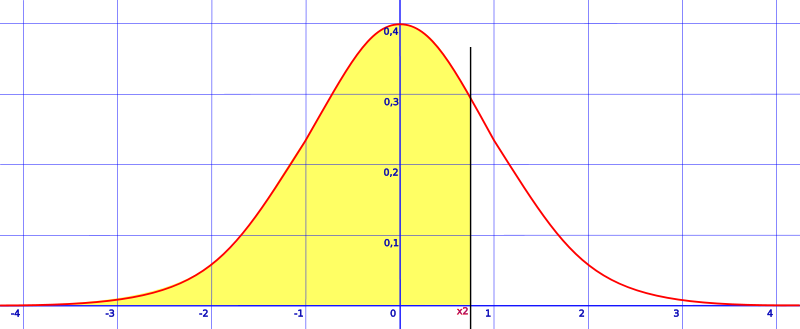# How to Find Area Left of a Z Score

Share on

You can find area to the left of a z score (where the z-score is greater than the mean) by using a z-table. The trick to using a z-table is knowing that the numbers represent percentages. For example, a value in the table of 0.5000 represents 50% of the area under the curve and a value of .9999 represents 99% of the area under a curve. Once you know how to read the table, finding the area only takes a step or two!

If you are looking for other variations on area, see the index article, Area Under a Normal Distribution Curve. You’ll find several articles for all different possibilities of areas. For example, finding the area for a value between 0 and any z-score, or an area to the right of a z-score.

## How to find area left of a z score: Steps

Watch the video for an example:Area shaded to the left of a z-score (z is greater than the mean).

Step 1: Split your given decimal into two after the tenths decimal place. For example, if you’re given 0.46, split that into 0.4 + 0.06.

Step 2: Look up your decimals from Step 1 in the z-table. The z-table below gives the result from looking up 0.4 in the left column and 0.06 in the top row. The intersection (i.e. the area under the curve) is .1772.

z 0.00 0.01 0.02 0.03 0.04 0.05 0.06 0.07 0.08 0.09
0.0 0.0000 0.0040 0.0080 0.0120 0.0160 0.0199 0.0239 0.0279 0.0319 0.0359
0.1 0.0398 0.0438 0.0478 0.0517 0.0557 0.0596 0.0636 0.0675 0.0714 0.0753
0.2 0.0793 0.0832 0.0871 0.0910 0.0948 0.0987 0.1026 0.1064 0.1103 0.1141
0.3 0.1179 0.1217 0.1255 0.1293 0.1331 0.1368 0.1406 0.1443 0.1480 0.1517
0.4 0.1554 0.1591 0.1628 0.1664 0.1700 0.1736 0.1772 0.1808 0.1844 0.1879
0.5 0.1915 0.1950 0.1985 0.2019 0.2054 0.2088 0.2123 0.2157 0.2190 0.2224

Step 3: Add 0.500 to the z-value you just found in step 2.

Note: You’re adding .500 because that’s the 50% of the graph between the mean at zero and the far left of the graph. The above steps only gave you a sliver between 0 and the z-score.

That’s it!

*note on How to find area left of a z score with negative values. The normal distribution is symmetrical, so if you are given negative values you can just look up their absolute values. For example, if you are asked for the area of a tail on the left to -0.96, look up the absolute value of -0.96 (0.96).

## References

Beyer, W. H. CRC Standard Mathematical Tables, 31st ed. Boca Raton, FL: CRC Press, pp. 536 and 571, 2002.
Klein, G. (2013). The Cartoon Introduction to Statistics. Hill & Wamg.
Lindstrom, D. (2010). Schaum’s Easy Outline of Statistics, Second Edition (Schaum’s Easy Outlines) 2nd Edition. McGraw-Hill Education
Wheelan, C. (2014). Naked Statistics. W. W. Norton & Company

CITE THIS AS:
Stephanie Glen. "How to Find Area Left of a Z Score" From StatisticsHowTo.com: Elementary Statistics for the rest of us! https://www.statisticshowto.com/probability-and-statistics/normal-distributions/how-to-find-area-left-of-a-z-score/
---------------------------------------------------------------------------Need help with a homework or test question? With Chegg Study, you can get step-by-step solutions to your questions from an expert in the field. Your first 30 minutes with a Chegg tutor is free!